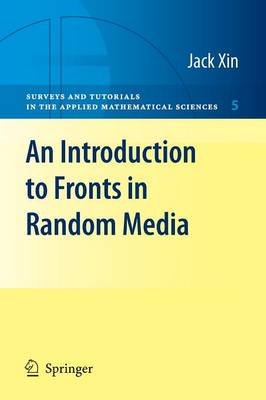•# An Introduction to Fronts in Random Media - Surveys and Tutorials in the Applied Mathematical Sciences 5 (Paperback)

(author)
£33.99
Paperback 162 Pages / Published: 13/07/2009
• We can order this

Usually dispatched within 3 weeks

This book aims to give a user friendly tutorial of an interdisciplinary research topic (fronts or interfaces in random media) to senior undergraduates and beginning grad uate students with basic knowledge of partial differential equations (PDE) and prob ability. The approach taken is semiformal, using elementary methods to introduce ideas and motivate results as much as possible, then outlining how to pursue rigor ous theorems, with details to be found in the references section. Since the topic concerns both differential equations and probability, and proba bility is traditionally a quite technical subject with a heavy measure theoretic com ponent, the book strives to develop a simplistic approach so that students can grasp the essentials of fronts and random media and their applications in a self contained tutorial. The book introduces three fundamental PDEs (the Burgers equation, Hamilton- Jacobi equations, and reaction-diffusion equations), analysis of their formulas and front solutions, and related stochastic processes. It builds up tools gradually, so that students are brought to the frontiers of research at a steady pace. A moderate number of exercises are provided to consolidate the concepts and ideas. The main methods are representation formulas of solutions, Laplace meth ods, homogenization, ergodic theory, central limit theorems, large deviation princi ples, variational principles, maximum principles, and Harnack inequalities, among others. These methods are normally covered in separate books on either differential equations or probability. It is my hope that this tutorial will help to illustrate how to combine these tools in solving concrete problems.

Publisher: Springer-Verlag New York Inc.
ISBN: 9780387876825
Number of pages: 162
Weight: 550 g
Dimensions: 235 x 155 x 9 mm
Edition: 2009 ed.